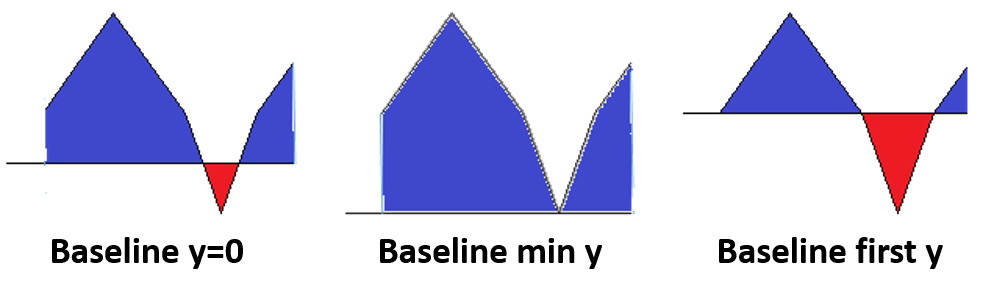Content DisclaimerCopyright @2020.All Rights Reserved.
StatsToDo : Area Under Coordinates
 Explanation Javascript ProgramThis page provides a calculation for the area under a set of coordinates, sometimes called Area Under the Curve (AUC) The area is calculated using 3 common models of AUC, as shown in the diagram to the right. The most commonly used model is the area above the line y=0. An example is a measurement of blood drug concentration (y value) over time after administration (x value), to estimate its biological activity Another common model is to use the first y value to draw the baseline, so that the area is that above this value. An example is the estimation of glucose tolerance, where the area above the fasting blood level is of interest The two models calculates the area above the line (blue area) as positive value, and area below the line (red area) as negative values, so that the final area is the difference (blue area - red area). The third model estimates the total positive area, and uses the minimum y value is the dataset as the baseline.